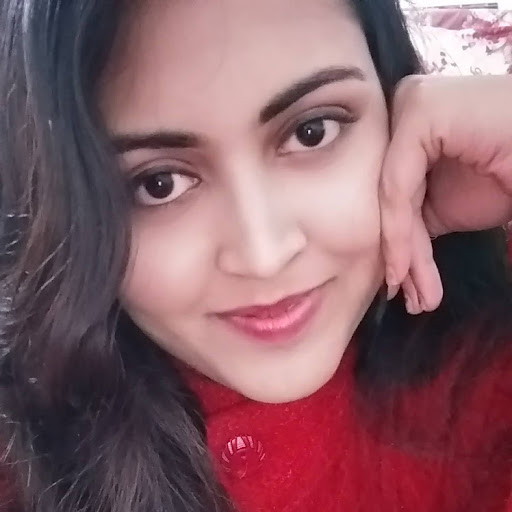# The price of 10 chairs is equal to that of 4 tables. The price of 15 chairs and 2 tables together is Rs. 4000. The total price of 12 chairs and 3 tables is:

A. Rs. 3500

B. Rs. 3750

C. Rs. 3840

D. Rs. 3900

E. None of these

### Solution(By Examveda Team)

\eqalign{ & {\text{Let}}\,{\text{the}}\,{\text{cost}}\,{\text{of}}\,{\text{a}}\,{\text{chair}}\,{\text{and}}\,{\text{that}}\,{\text{of}}\,\,{\text{table}} \cr & \,{\text{be}}\,Rs.\,x\,{\text{and}}\,Rs.\,y\,{\text{respectively}}. \cr & {\text{Then}},\,10x = 4y\,\,\,or\,\,\,y = \frac{5}{2}x \cr & \therefore 15x + 2y = 4000 \cr & \Rightarrow 15x + 2 \times \frac{5}{2}x = 4000 \cr & \Rightarrow 20x = 4000 \cr & \therefore x = 200 \cr & {\text{So}},\,y = {\frac{5}{2} \times 200} = 500 \cr & {\text{Hence,}}\,{\text{the}}\,{\text{cost}}\,{\text{of}}\,{\text{12}}\,{\text{chairs}}\,{\text{and}}\,{\text{3}}\,{\text{tables}} \cr & = 12x + 3y \cr & = Rs.\,\left( {2400 + 1500} \right) \cr & = Rs.\,3900 \cr}

1.4T=10C
2T=5C
ATQ,15C+2T=4000
OR,15C+5C=4000
OR,C=200
2T=5C
T=5C/2=5*200/2=500
12C+3T=12*200+3*500=3900

2.Price of 10chair=price of 4tables
Or, 10C= 4T
C/T= 4/10=2/5 or 2:5
15x2+2x2=40
if 40units= 4000rupees
2 units= 200 rupees
5 units = 500rupees

3.The price of 12 chairs is equal to that of 5 tables. The price of 8 chairs and 3 tables together is rs.5700. The total price of 9 chairs and 6 tables is

4.10ch=4tb
15ch=4/10*15=6table
6tb+2tb price 4000tk
1tb price 500tk
3tb price 500*3=1500......

10ch=4tb
12ch=4/10*12=4.8tb
500*4.8=2400......
1500+2400=3900

Related Questions on Simplification